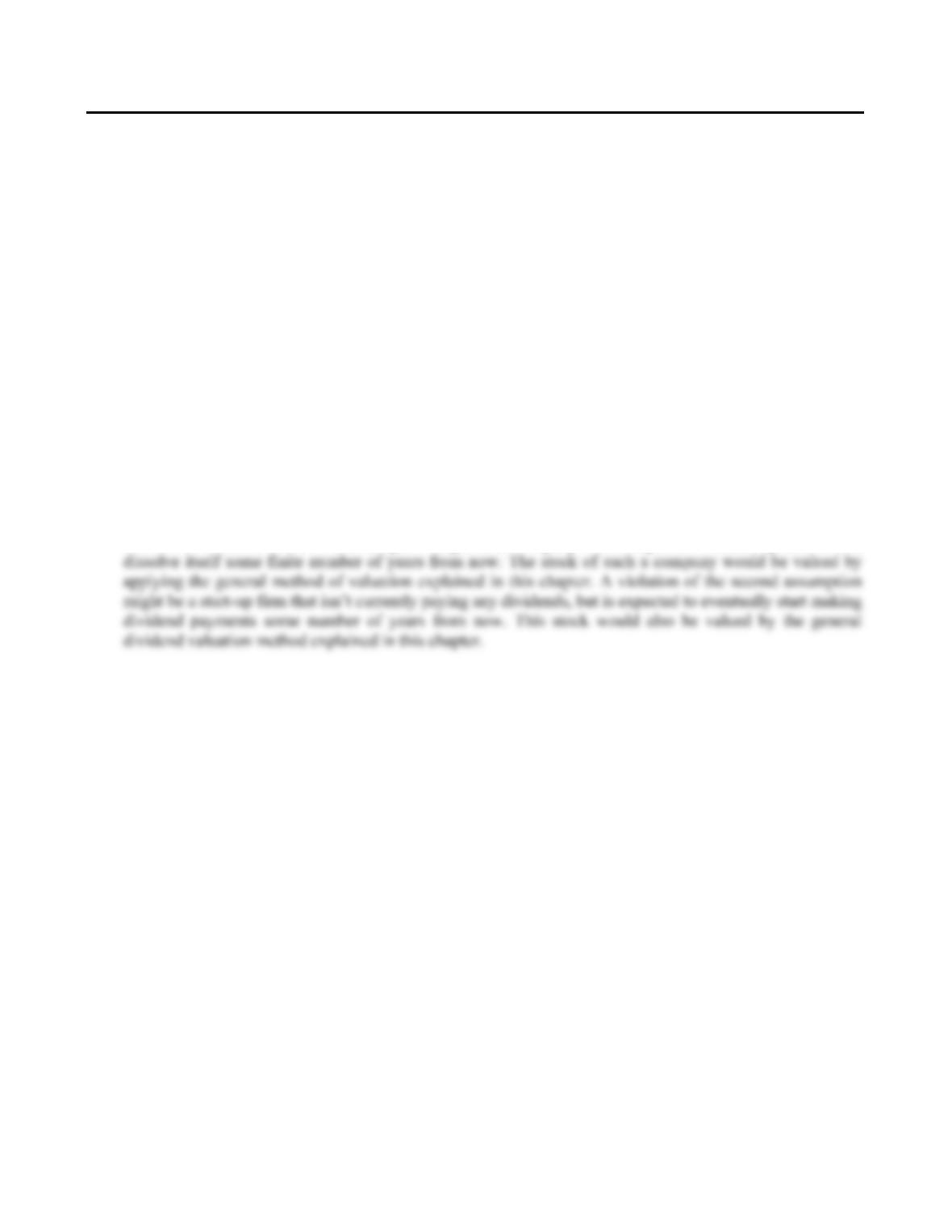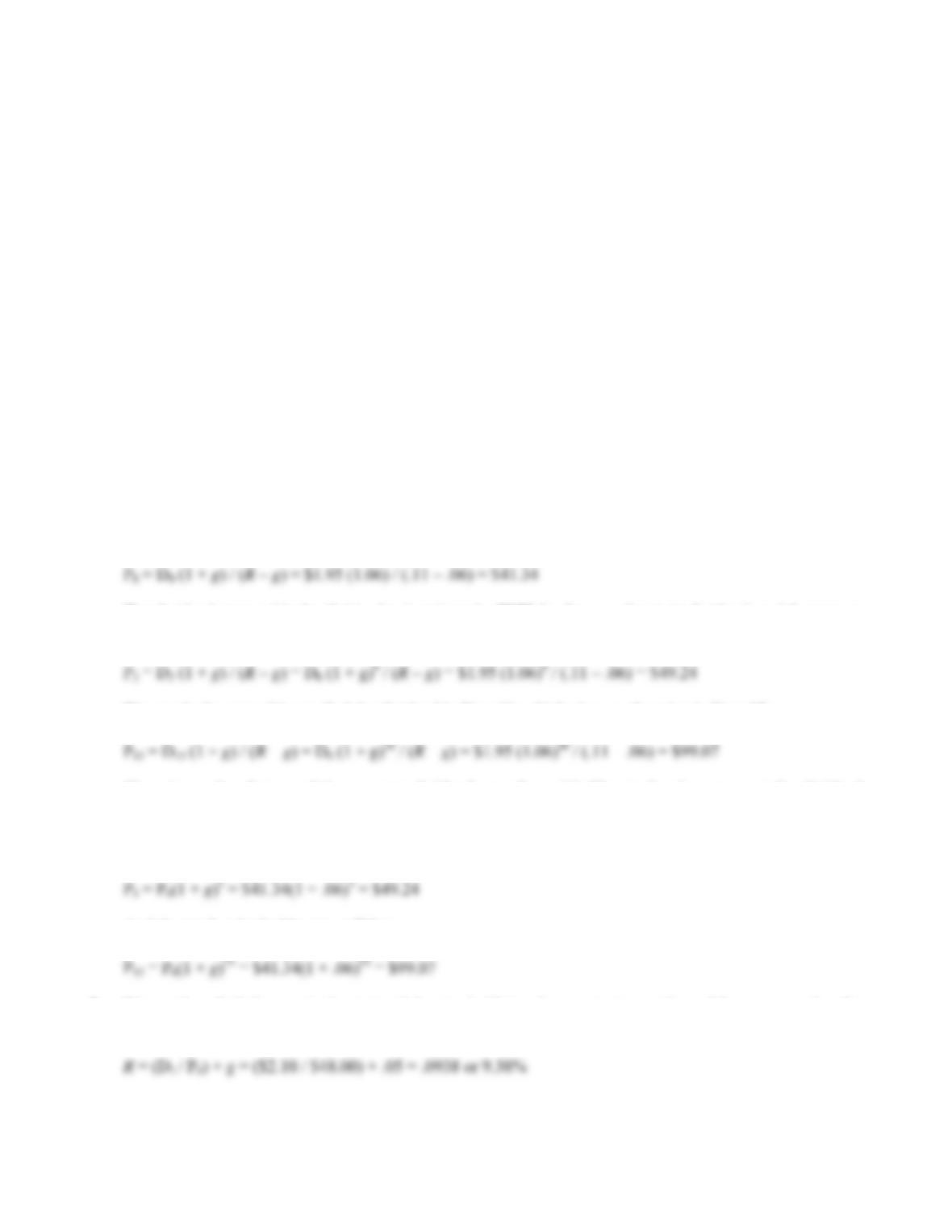Type
Quiz
Book Title
Fundamentals of Corporate Finance Standard Edition 9th Edition
ISBN 13
978-0073382395

### 978-0073382395 Chapter 8 Concepts Review and Critical Thinking Questions

April 3, 2019CHAPTER 8
STOCK VALUATION
Answers to Concepts Review and Critical Thinking Questions
1. The value of any investment depends on the present value of its cash flows; i.e., what investors will
actually receive. The cash flows from a share of stock are the dividends.
2. Investors believe the company will eventually start paying dividends (or be sold to another company).
3. In general, companies that need the cash will often forgo dividends since dividends are a cash expense.
Young, growing companies with profitable investment opportunities are one example; another example is a
company in financial distress. This question is examined in depth in a later chapter.
4. The general method for valuing a share of stock is to find the present value of all expected future dividends.
The dividend growth model presented in the text is only valid (i) if dividends are expected to occur forever,
that is, the stock provides dividends in perpetuity, and (ii) if a constant growth rate of dividends occurs
forever. A violation of the first assumption might be a company that is expected to cease operations and
5. The common stock probably has a higher price because the dividend can grow, whereas it is fixed on the
preferred. However, the preferred is less risky because of the dividend and liquidation preference, so it is
possible the preferred could be worth more, depending on the circumstances.
6. The two components are the dividend yield and the capital gains yield. For most companies, the capital
gains yield is larger. This is easy to see for companies that pay no dividends. For companies that do pay
dividends, the dividend yields are rarely over five percent and are often much less.
7. Yes. If the dividend grows at a steady rate, so does the stock price. In other words, the dividend growth rate
and the capital gains yield are the same.
8. In a corporate election, you can buy votes (by buying shares), so money can be used to influence or even
determine the outcome. Many would argue the same is true in political elections, but, in principle at least,
no one has more than one vote.
9. It wouldn’t seem to be. Investors who don’t like the voting features of a particular class of stock are under
no obligation to buy it.
10. Investors buy such stock because they want it, recognizing that the shares have no voting power.
Presumably, investors pay a little less for such shares than they would otherwise.B-142 SOLUTIONS
11. Presumably, the current stock value reflects the risk, timing and magnitude of all future cash flows, both
short-term and long-term. If this is correct, then the statement is false.
12. If this assumption is violated, the two-stage dividend growth model is not valid. In other words, the price
calculated will not be correct. Depending on the stock, it may be more reasonable to assume that the
dividends fall from the high growth rate to the low perpetual growth rate over a period of years, rather than
in one year.
Solutions to Questions and Problems
NOTE: All end of chapter problems were solved using a spreadsheet. Many problems require multiple steps.
Due to space and readability constraints, when these intermediate steps are included in this solutions manual,
rounding may appear to have occurred. However, the final answer for each problem is found without rounding
during any step in the problem.
Basic
1. The constant dividend growth model is:
P
t = Dt × (1 + g) / (Rg)
So the price of the stock today is:
P
The dividend at year 4 is the dividend today times the FVIF for the growth rate in dividends and four years,
so:
P
We can do the same thing to find the dividend in Year 16, which gives us the price in Year 15, so:
P
There is another feature of the constant dividend growth model: The stock price grows at the dividend
growth rate. So, if we know the stock price today, we can find the future value for any time in the future we
want to calculate the stock price. In this problem, we want to know the stock price in three years, and we
have already calculated the stock price today. The stock price in three years will be:
P
And the stock price in 15 years will be:
P
2. We need to find the required return of the stock. Using the constant growth model, we can solve the
equation for R. Doing so, we find: